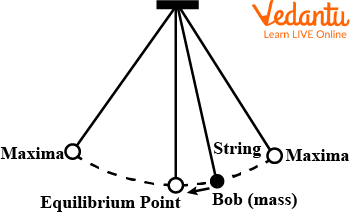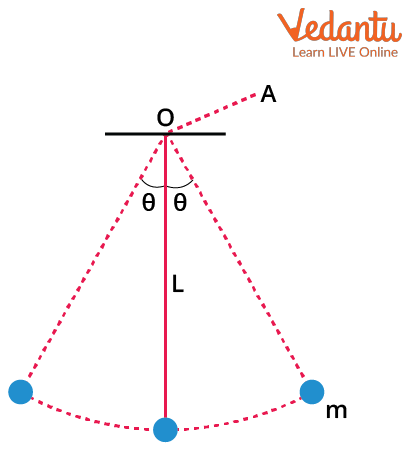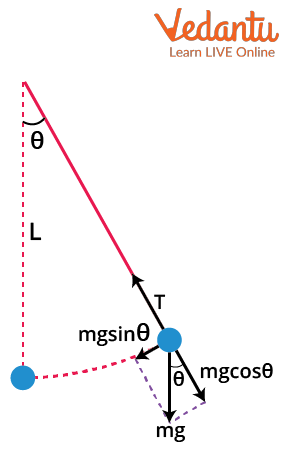Courses
Courses for Kids
Free study material
Free LIVE classes
More

# Time Period of Simple Pendulum for JEELIVE
Join Vedantu’s FREE Mastercalss

## Simple Pendulum: A Mechanical Device

A simple pendulum is a mechanical device with a periodic motion of a little circular ball hanging from any fixed end by a thin indivisible string of length L. It is on a vertical plane and is propelled by a gravity pull, which causes the oscillations to travel. A massless ball hangs at the end of a rope. The length of the string must be increased to increase the time.

The period of a basic pendulum is generally determined by the pendulum's position and acceleration due to gravity, which varies across the globe. Any pendulum's period is independent of the suspended sphere's mass. In most cases, a point mass is attached to an unbreakable and light string suspended from continuous support, forming a simple pendulum. A single resonant frequency exists in the pendulum.

## What is a Simple Pendulum?

A simple pendulum is a device in which the point mass is suspended from fixed support and is attached to a light inextensible thread. The mean position of a simple pendulum is the vertical line passing through the fixed support. The length of the simple pendulum, represented by L, is the vertical distance between the point of suspension and the suspended body's centre of mass (when it is in the mean position). The pendulum in this form is based on a resonant system with a single resonant frequency.

### Simple Pendulum DiagramImage: Simple Pendulum Diagram

In the above image, we can see a simple pendulum where a string is fixed at one end and on the other, a mass called ‘bob’ is attached to it. The central position of the bob or the rest position is called the ‘equilibrium point’ and the maximum distance up to which it oscillates is called its ‘maxima’.

### The Motion of a Pendulum

The pendulum starts consonant movement when a point uproots the sway. For little upsides of a dislodging end, the symphonious movement is a basic consonant movement with precise relocation of the bounce. When the wavering of a short pendulum is slight or the sufficiency of the swaying is tiny in contrast with the two lengths of the string, it might at any point be respected as a basic symphonious movement. The movement of a short pendulum is accepted to be a basic symphonious movement utilising a biassed point estimate.

### Time Period of Simple Pendulum

The amount of time necessary to complete an oscillation in a particular period is the period from which we can calculate the frequency for which we have angular velocity. The restoring force and period can be calculated if the provided object moves in a simple harmonic motion. The time necessary for a simple pendulum to accomplish one complete oscillation, which begins at its average position and ends at its middle class after completing one total change, is known as the ‘period of the simple pendulum’.

### Calculation of a Simple Pendulum's Time Period

For the calculation of the time period of a simple pendulum, it is very important to comply with some conditions, which are as follows:

• The arms of a basic pendulum must be rigid and massless to determine the time period.

• A frictionless environment is required for the time period of a simple pendulum.

• The pendulum's motion should be in a precise plane.

• Gravitational acceleration must also be constant.Image: A Simple Pendulum and the Axis of Rotation

When the bob of the pendulum is displaced from its mean position, it starts oscillating in a circular arc about it. We could say that the particle makes a pure rotation about the horizontal axis OA which is perpendicular to the plane of the paper and perpendicular to the plane of motion.Image: Freebody Diagram of the Pendulum

Now from the Freebody diagram of the pendulum, we can solve it.

We can employ the motion equation. The tension in the string is balanced by mgcos$\theta$. The equation will be:

\begin{align} &T-m g \cos \theta=0 \\ &T=m g \cos \theta \end{align}

Torque $\tau$ is used to bring the mass to its equilibrium position. This torque is equal to $mg\sin{\theta}$ which is clear from the diagram. Now, the torque is given by the product of force and the distance of the point of application of force from the axis of rotation. In this case, the distance is equal to L which is the length of the string.

So, we can write the equation that

\begin{align} &\tau=m g \sin \theta \times L \\ &\tau=m g l \sin \theta \end{align}

Since this torque tries to bring the particle back to $\theta=0$, it will be negative and so we can write,

$\tau=-m g L \sin \theta$

We know that

$\tau=I \alpha$

Here, I is the moment of inertia and α is the angular acceleration.

For oscillations with small angles,

$\sin{\theta}=\theta$

So, we can write that

\begin{align} &I \alpha=-m g L \theta \\ &\alpha=-\dfrac{m g L \theta}{I} \end{align}

The moment of inertia for this bob about the axis OA would be

I=mL2

This gives

\begin{align} &\alpha=-\dfrac{m g L \theta}{m L^{2}} \\ &\alpha=-\dfrac{g}{L} \theta \end{align}

Also we can write

$\alpha=-\omega^{2} \theta$

This means that

\begin{align} &\omega^{2}=\dfrac{g}{L} \\ &\omega=\sqrt{\dfrac{g}{L}} \end{align}

So, we can now write the time period T of the simple pendulum as:

\begin{align} &T=\dfrac{2 \pi}{\omega} \\ &T=2 \pi \sqrt{\dfrac{L}{g}} \end{align}

Here, we got the formula of the time period for a simple pendulum,

where

T= Period in Seconds,

m= mass of the pendulum,

g = Gravitational acceleration = 9.80665 $\dfrac{m}{s^2}$ on Earth,

L= The length of the rod or wire(m or ft),

M = Moment of the inertia of the Bob, and

$\theta$ = Small Angle.

### Simple Pendulum g Formula

Pendulums are used for a variety of purposes, including clocks, and a swing in the garden is likewise similar to a pendulum. The following is the formula in regards to this:

According to the formula of the time period we have,

Time Period $=\dfrac{2 \Pi}{\omega_{0}}=2 \Pi \times \sqrt{\dfrac{L}{g}}$

Potential Energy $=m g l(1-\cos \theta)$

Kinetic Energy $=\dfrac{1}{2} m v^{2}$

Total Energy = Potential energy + Kinetic Energy

$=\dfrac{2\Pi}{\omega_{0}}=2\Pi\times\sqrt{\dfrac{L}{g}}+\dfrac{1}{2}m v^{2}$.

where

V = Velocity of the pendulum and $\omega_0=$ Angular Frequency of the Pendulum.

### Application of Simple Pendulum

Simple pendulums are utilised in tickers as the pendulum makes some decent memories and they can be used to monitor time. Following is an illustration of a simple pendulum:

• Pendulums are used to determine how much speed increases due to gravity.

• A metronome can be made out of a pendulum.

### Solved Example on Simple Pendulum's Time Period

1. A Simple Pendulum has a period of 1.2 seconds. What is the pendulum's length? $\left(g=10~\dfrac{m}{s^{2}}\right)$

Solution:

Given here,

Simple Pendulum has a period of 1.2 seconds

And $g=10~\dfrac{m}{ s^{2}}$

Time Period $=\dfrac{2 \Pi}{\omega_{0}}=2 \Pi \times \sqrt{\frac{L}{g}}$

\begin{align} &1.2=2 \Pi \times \sqrt{\dfrac{L}{10}} \\ & L=0.36~m \end{align}

The length of the pendulum will be 0.36 m.

2. On the surface of the Moon, gravity causes an acceleration of 1.8 m/s2. What is the period of a simple pendulum on the Moon if it has a 3.6-second period on Earth?

Solution:

Given here,

$g=1.8~\dfrac{m}{ s^{2}}$

Time period on earth T = 3.6 seconds

To find,

Time Period $=\dfrac{2 \Pi}{\omega_{0}}=2 \Pi \times \sqrt{\dfrac{L}{g}}$

\begin{align} & T=\dfrac{2 \Pi}{\omega_{0}} \\ &T=211 \times \sqrt{\frac{L}{10}} \\ &L=3.2 \mathrm{~m} \end{align}

On the moon,

\begin{align} & T=\dfrac{2 \pi}{\omega_{0}} \\ & T=2 \Pi \times \sqrt{\dfrac{3.2}{1.8}} \\ & T=8.17~sec \end{align}

As a result, the pendulum on the Moon has a time period of 8.17 seconds.

### Conclusion

A simple pendulum is a mechanical mechanism that oscillates or moves back and forth. The force of gravity is the primary driver of this motion, which takes place in a vertical plane. The remarkable thing is that the ball suspended at the end of a thread is extremely light, almost massless. In this article, we discussed the simple pendulum time period and also explained how to calculate the same. Simple pendulum formula can be used to calculate the time period of its oscillation.

Last updated date: 24th Sep 2023
Total views: 130.8k
Views today: 2.30k

## FAQs on Time Period of Simple Pendulum for JEE

1. What is the definition of a simple pendulum? What makes a simple pendulum different from a compound pendulum?

A simple pendulum is an object with a small mass (pendulum bob) hanging on a light wire or thread. The pendulum bob oscillates on a plane around the vertical line via the support when it is shifted. The length of a simple pendulum is the only physical property that influences its period. The period is unaffected by the object's mass. The period of a compound pendulum is determined by the object's mass and how that mass is distributed.

2. What are the three parts that make up a pendulum? What effect does gravity have on a pendulum?

A pendulum comprises only a few parts: a length of thread or wire, a bob or other weight, and a fixed point. They can be used to demonstrate that the earth spins on its axis. Gravity is a constant force that pushes the pendulum downward, whether at rest or in motion. The pendulum swings side-to-side in a circular motion, thanks to gravity. The tension force owing to the string that binds the weight is the other force operating on the pendulum.